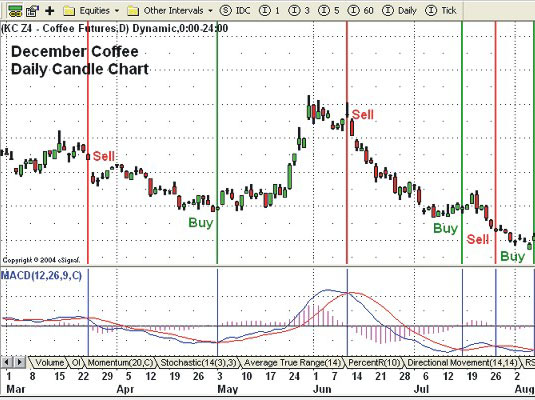# Moving Average Convergence/Divergence (MACD)

Gerald Appel developed an interesting oscillator that provides greater emphasis on recent price action over more distant activity through a creative use of exponential moving averages. The first step in the derivation of this indicator is to calculate a 12 and a 26 day exponential moving average from your price data. Your next step would be to take the difference of these two averages to calculate a MACD value. This line is plotted on your chart as the MACD Line. The Signal Line is next calculated by taking a 9 period exponential moving average of the MACD Line. Hence, the values of (12, 26, 9, C) on our study graph. Thus, you have a volatile line being crossed by a slower, less volatile line.

The use of this graph is similar in many ways to the use of a moving average crossover indicator. When the faster MACD Line crosses above the slower Signal Line you will look for opportunities to go long the market. A crossing of the MACD Line below the Signal Line is a sell signal. As with most oscillators, an extreme high value is indicative of an overbought market. An extreme low value represents an oversold market. You can also look for bullish or bearish divergences with the price action. An interesting presentation of the data is when you plot the difference between the MACD and Signal Lines to create a MACD Histogram. The Histogram goes through the zero level whenever the MACD and Signal Lines cross. Therefore, a sell signal is generated whenever the MACD Histogram falls below zero and a buy signal whenever it rises above zero. Conservative traders will often use a leveling or reduction in the slope of the histogram as an advance signal of a trend change to offset positions and take profits.